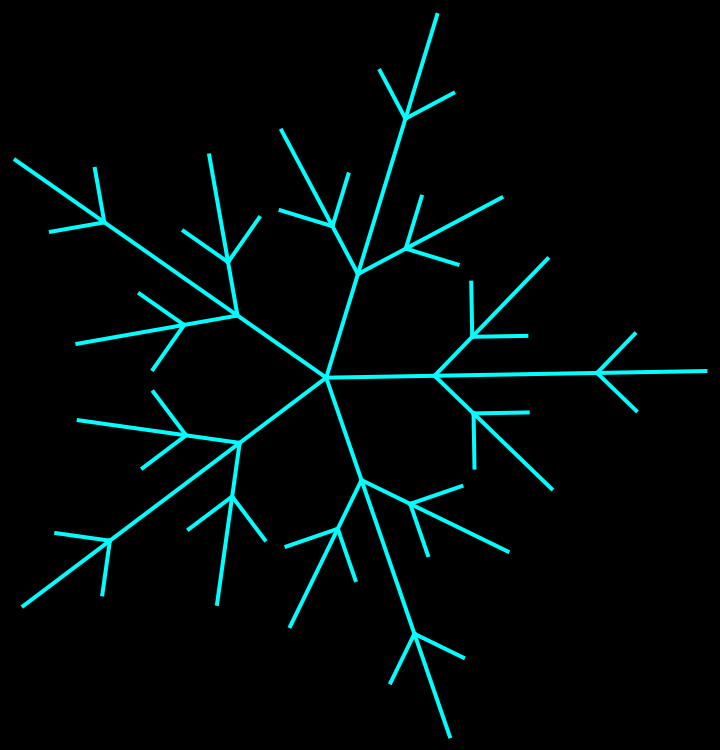#Function Repository Resource:

# LSystem

Generate an L-system

Contributed by: Renan Soares Germano
 ResourceFunction["LSystem"][rules,init,t,θ] returns an Association containing the word and the branches generated after executing the L-system represented by rules from initial word init for t steps, with movement specified by the angle θ.

## Details and Options

L-system is also known as a Lindenmayer system.
θ is taken to be in degrees.
The following special symbols, when present in rules, represent the following instructions:
 + increase the current angle by θ - decrease the current angle by θ [ start a new branch ] close the current branch
ResourceFunction["LSystem"] takes the following options:
 "InitialAngle" 90 initial angle in degrees from which the next points will be generated "InitialPoint" {0,0} intial point from which the next points will be generated "MoveLength" 1 distance of a new point from the previous one
Any other symbol will be interpreted as an instruction to move forward.
In an L-system with brackets, every left bracket "[" must be paired with a right bracket "]" in the rules and the initial word.

## Examples

### Basic Examples (4)

Run a simple L-system for one iteration:

 In:=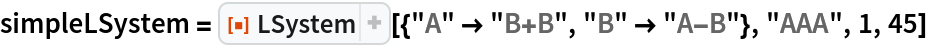Out=Because this L-system doesn't use brackets, it contains only one branch:

 In:=Out=Run a simple L-system with brackets for one iteration:

 In:=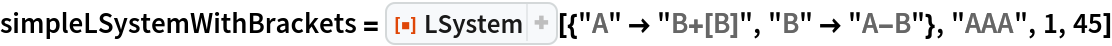Out=Because this L-system uses brackets, it contains multiple branches:

 In:=Out=Show a fractal:

 In:=Out=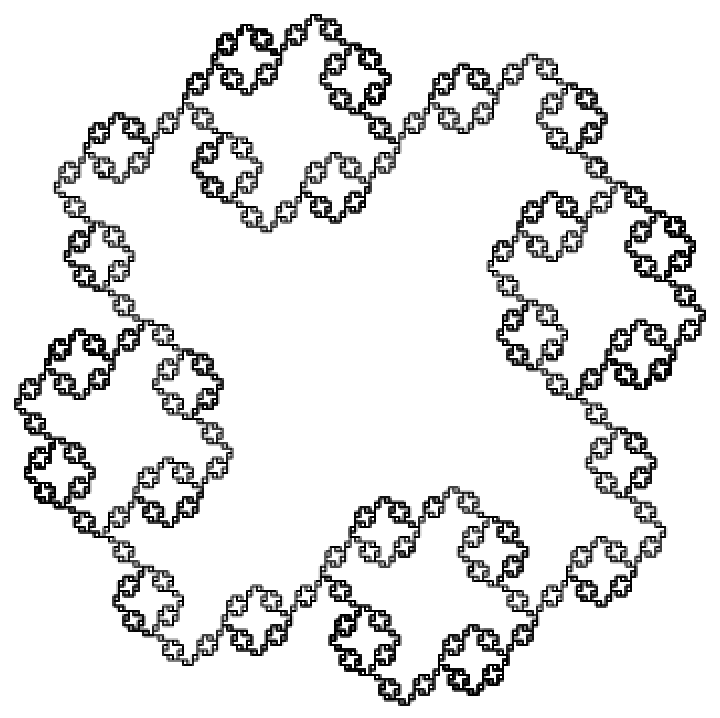Show a tree:

 In:=Out=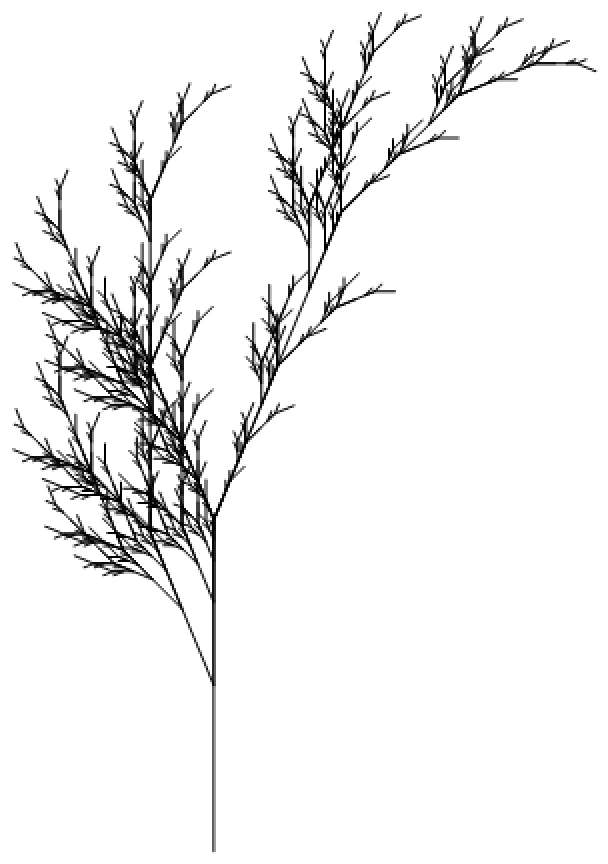### Scope (2)

Display a variety of fractals:

 In:=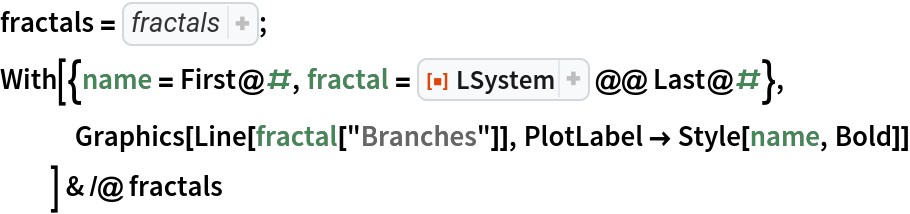Out=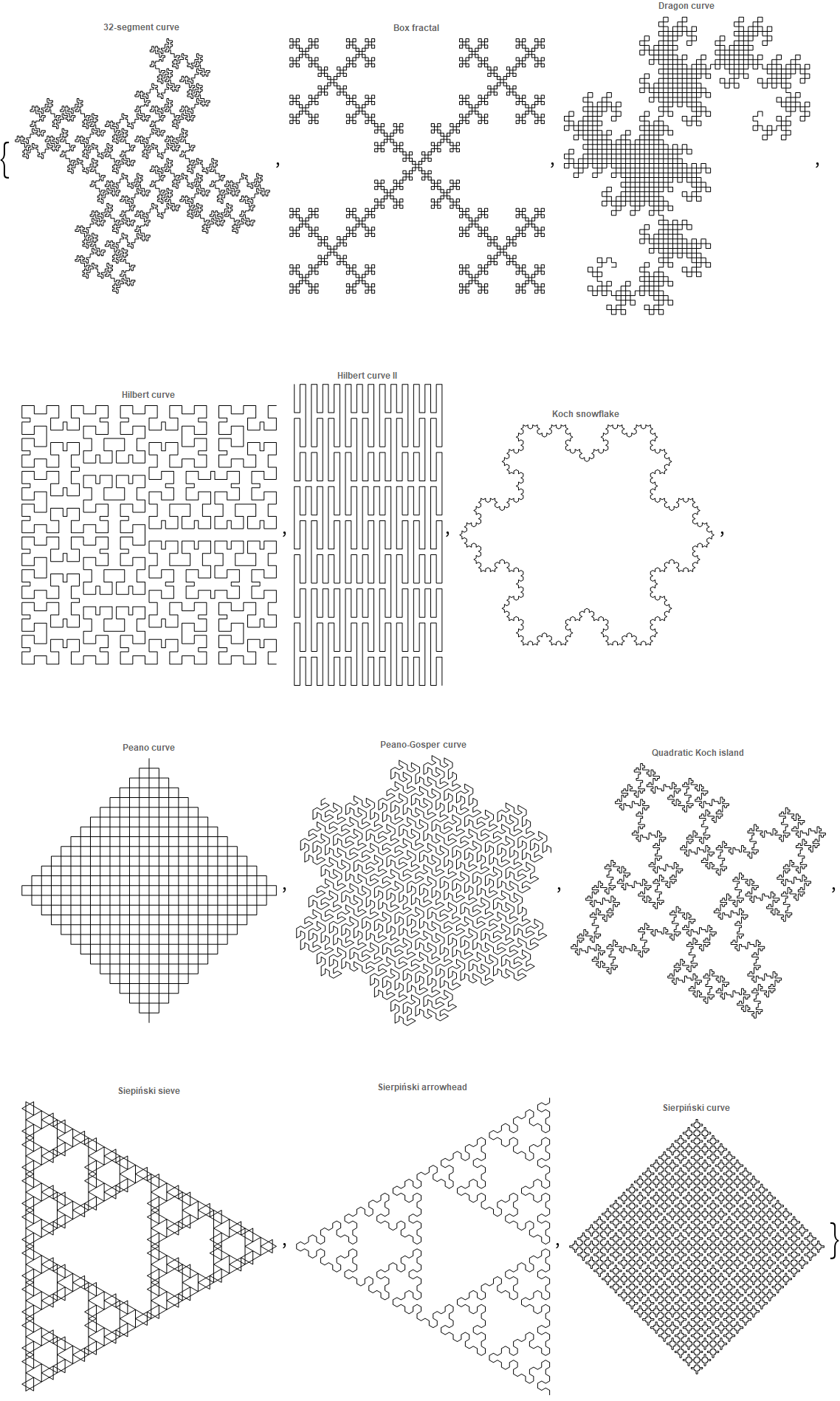Plot different trees:

 In:=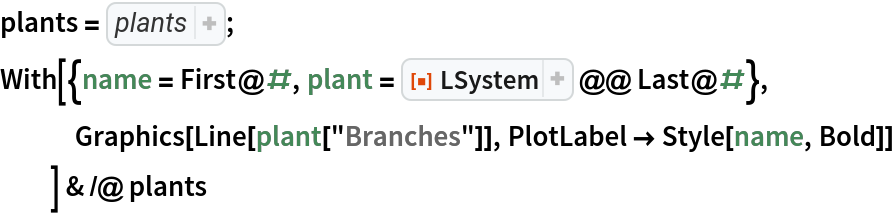Out=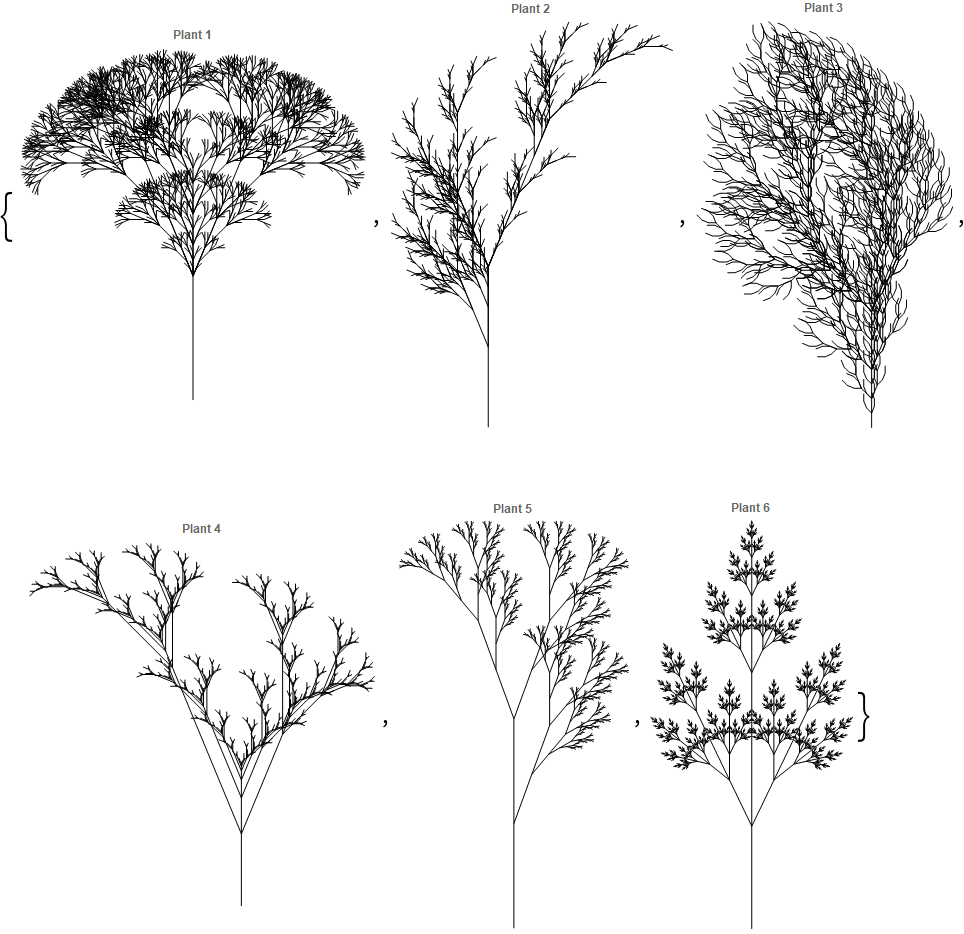### Options (3)

#### InitialAngle (1)

Show the effect of changing the initial angle:

 In:=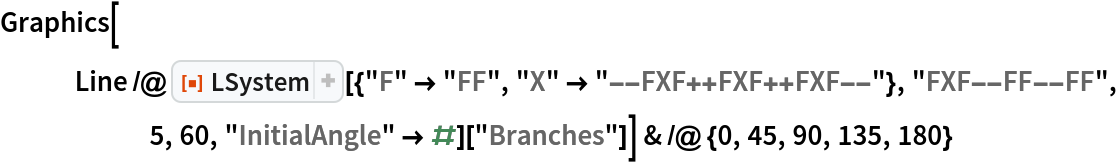Out=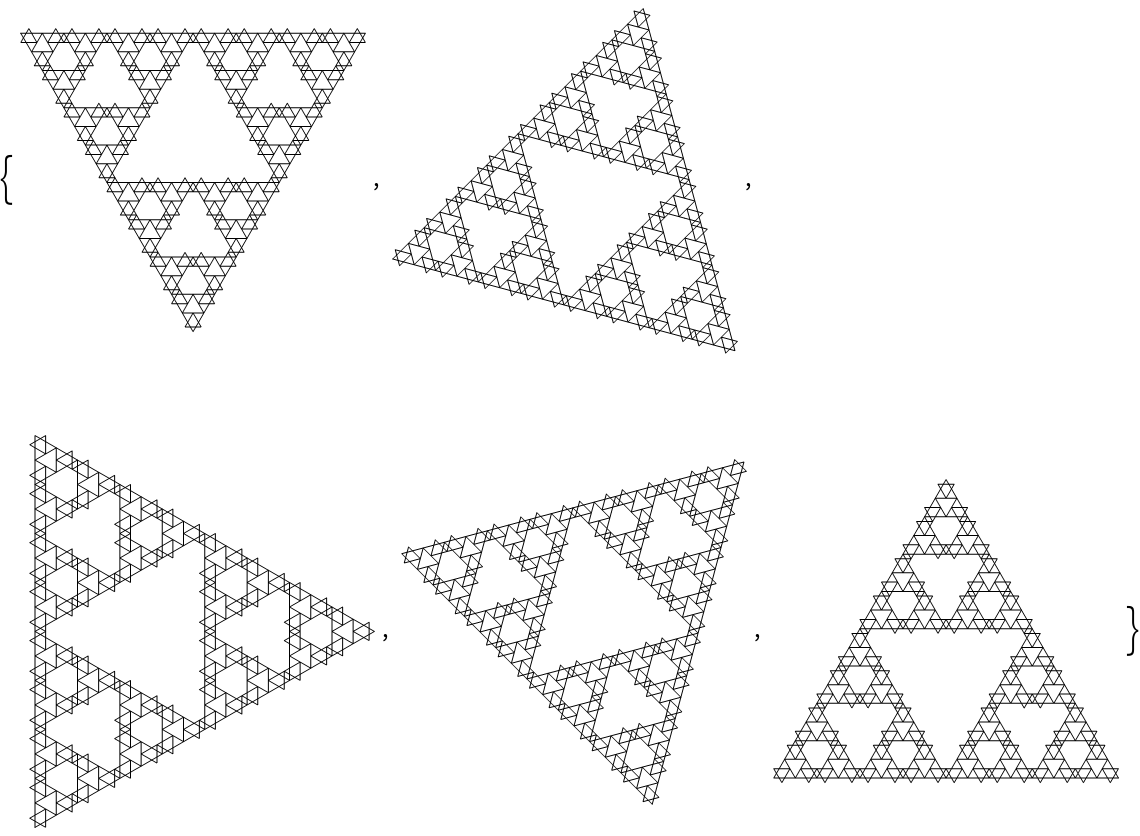In:=Out=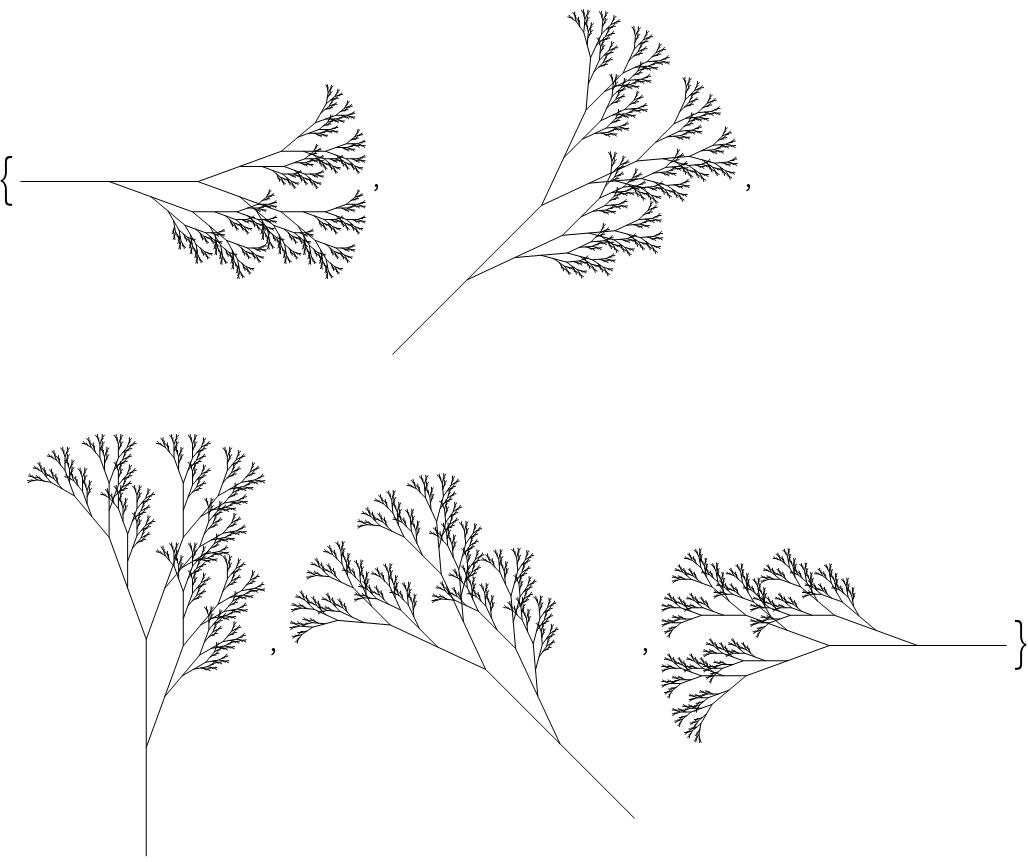#### InitialPoint (1)

Show the effect of changing the initial point:

 In:=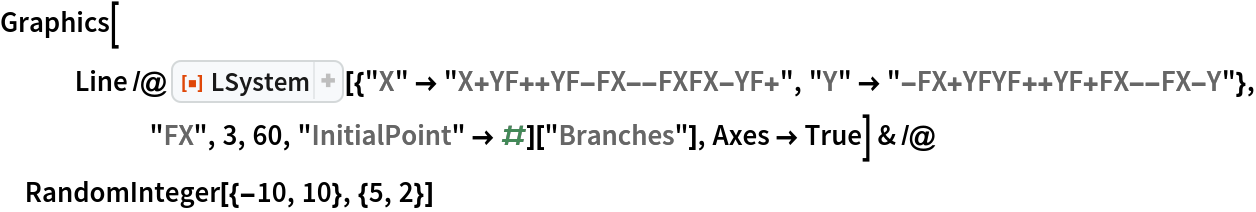Out=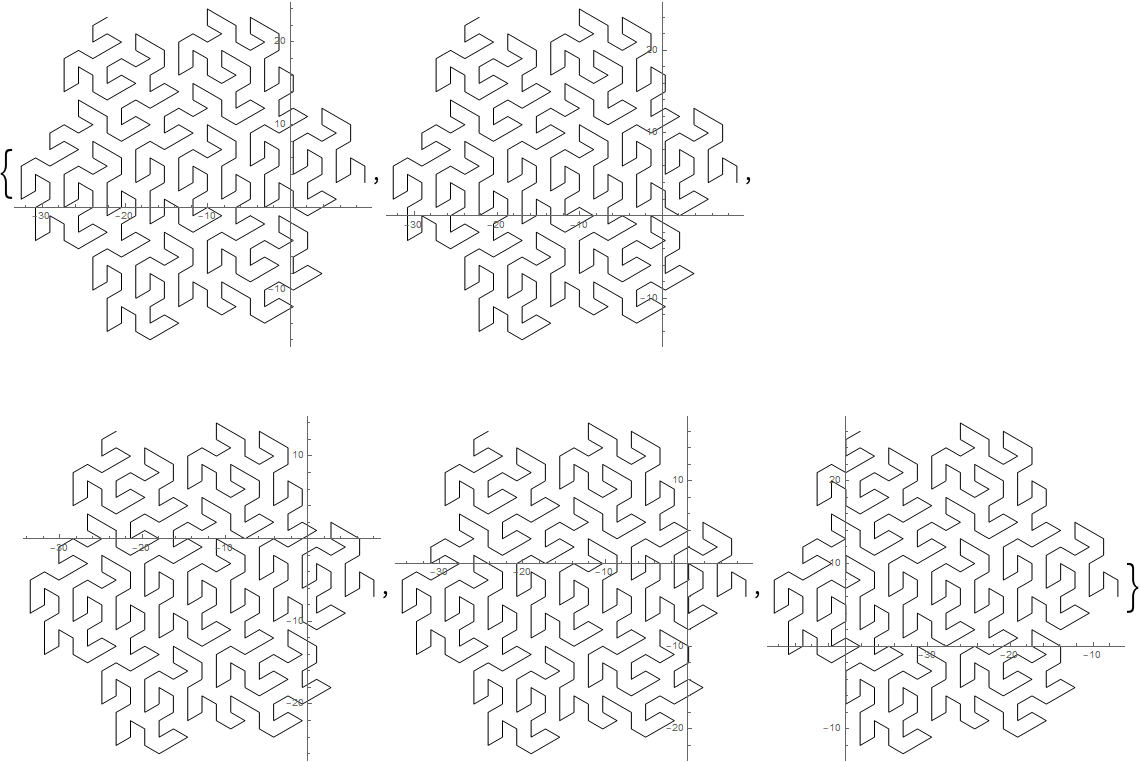In:=Out=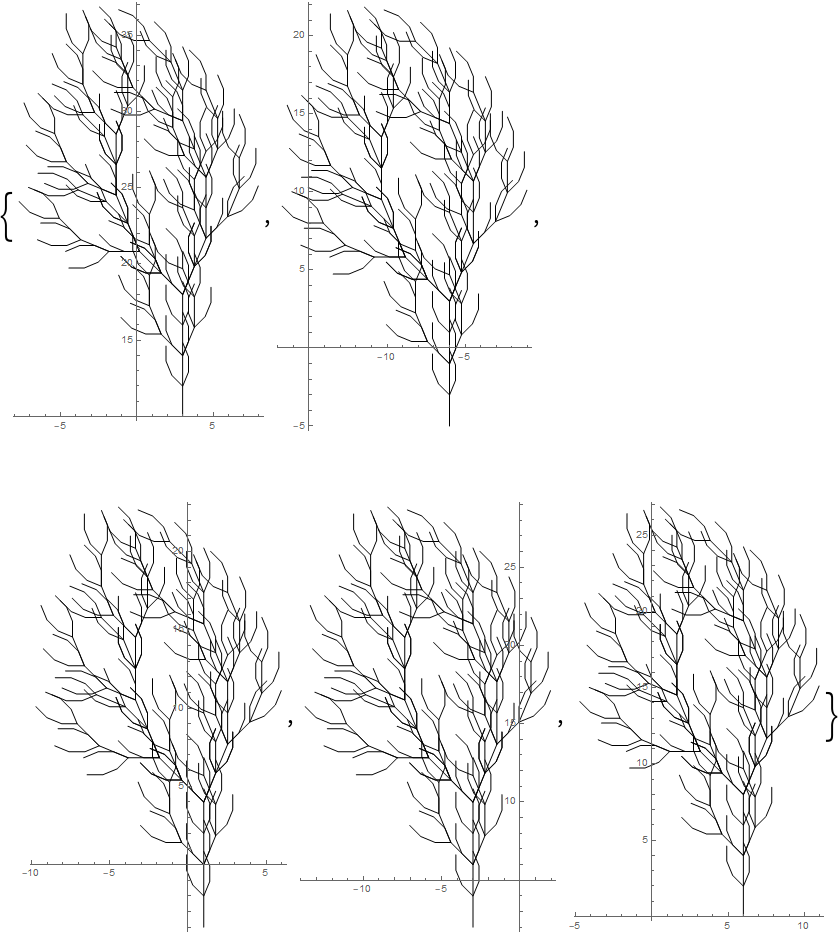#### MoveLength (1)

Show the effect of using different values for "MoveLength":

 In:=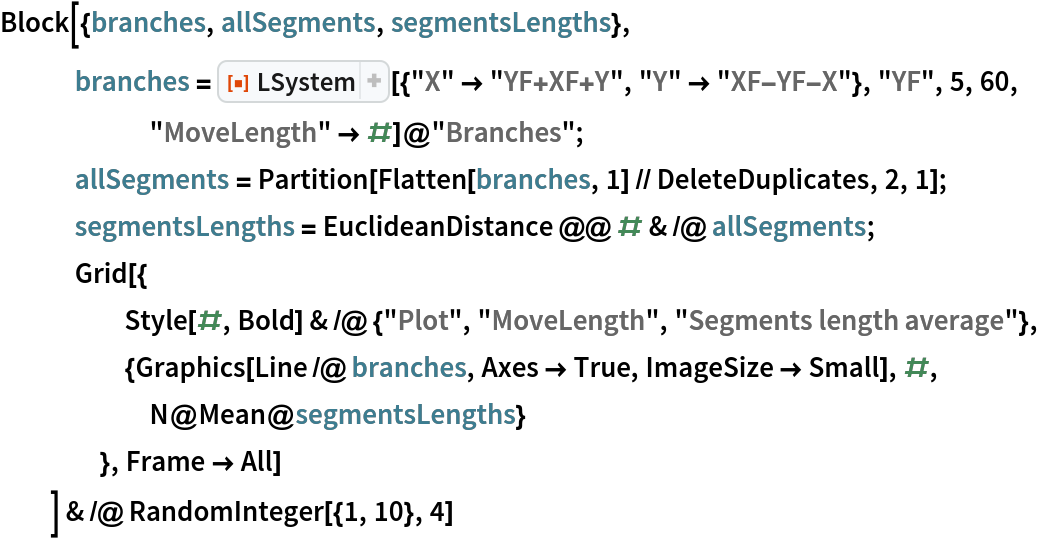Out=### Applications (6)

Show the step-by-step creation of the dragon curve:

 In:=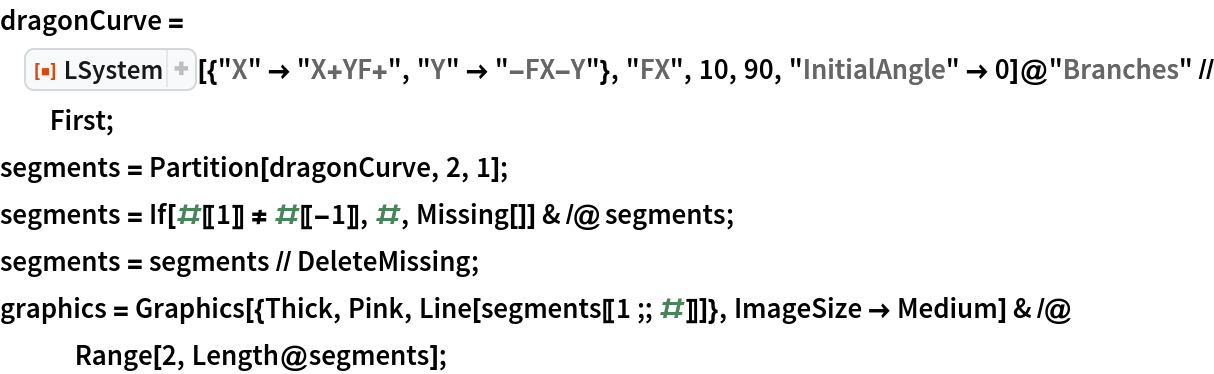In:=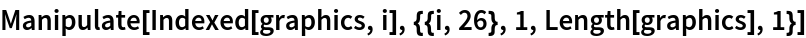Out=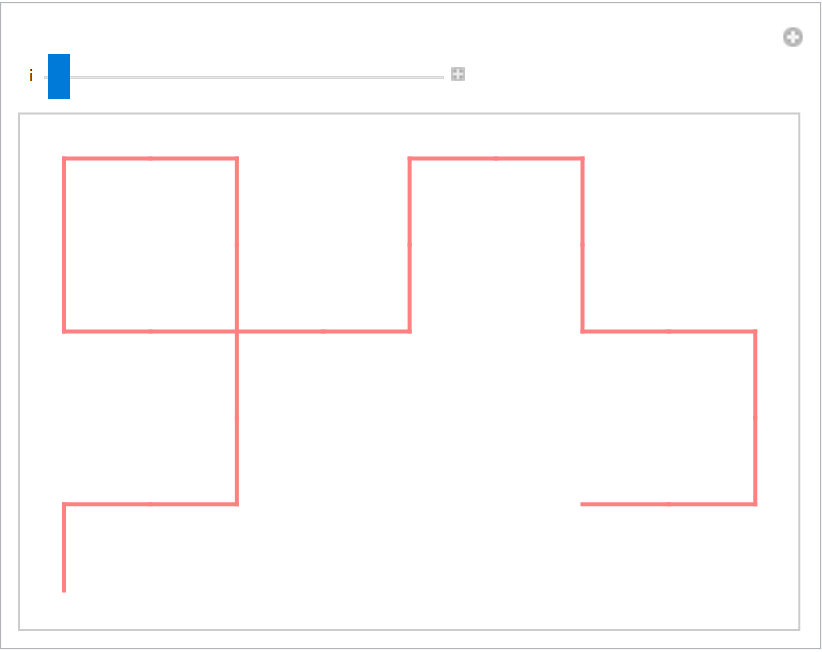Show the step-by-step creation of the Peano curve:

 In:=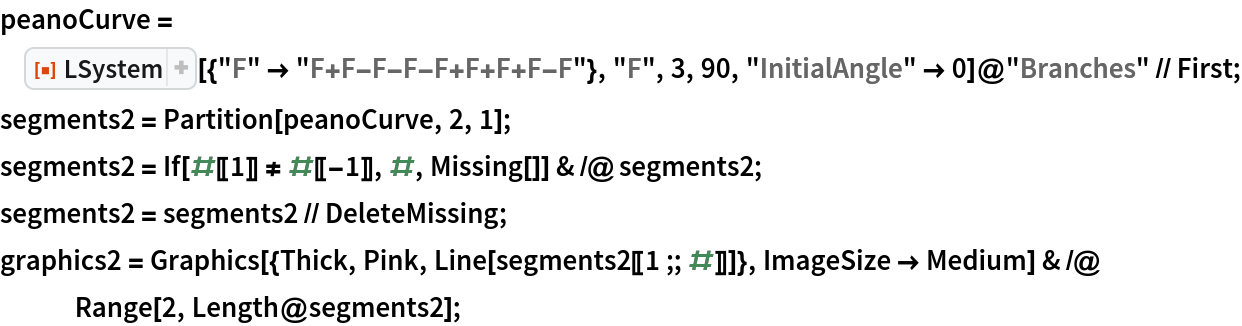In:=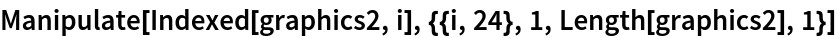Out=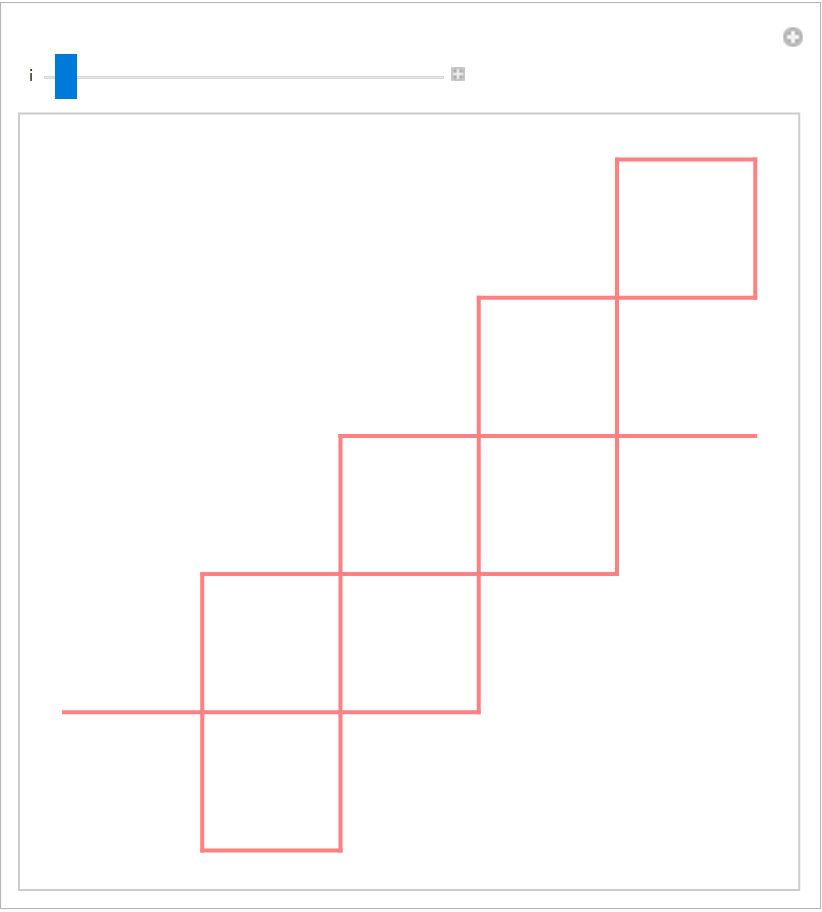Create a colorful tree:

 In:=Out=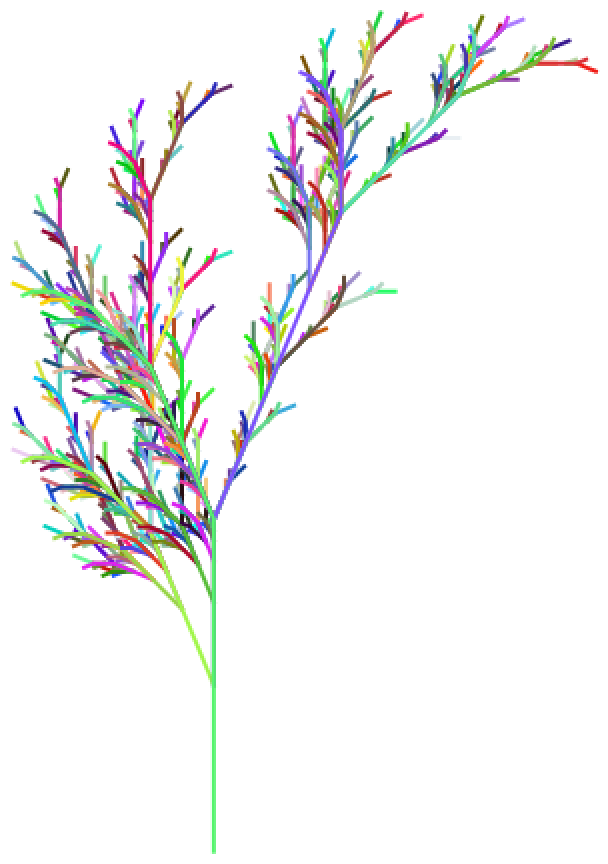Create a tree with green leaves:

 In:=Out=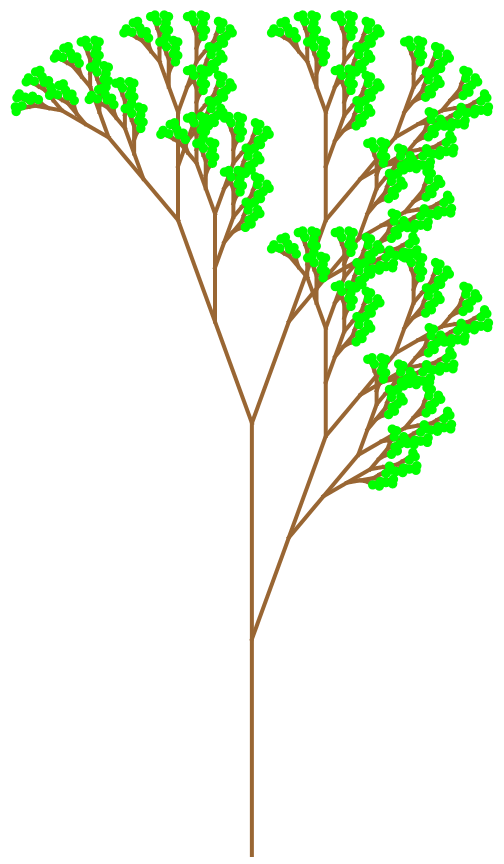Create a colorful plant with leaves:

 In:=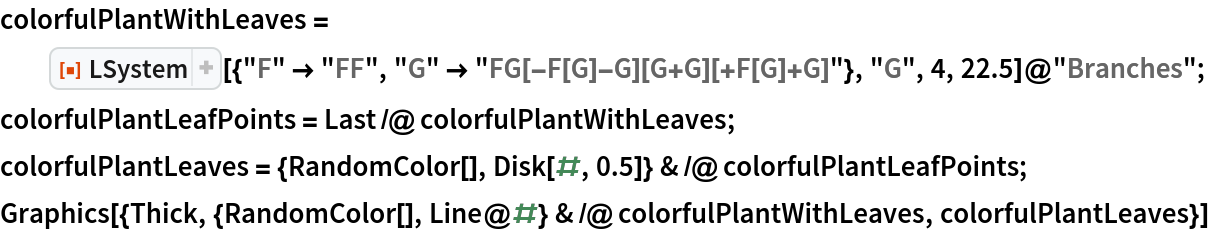Out=Create a colorful tree with grapes:

 In:=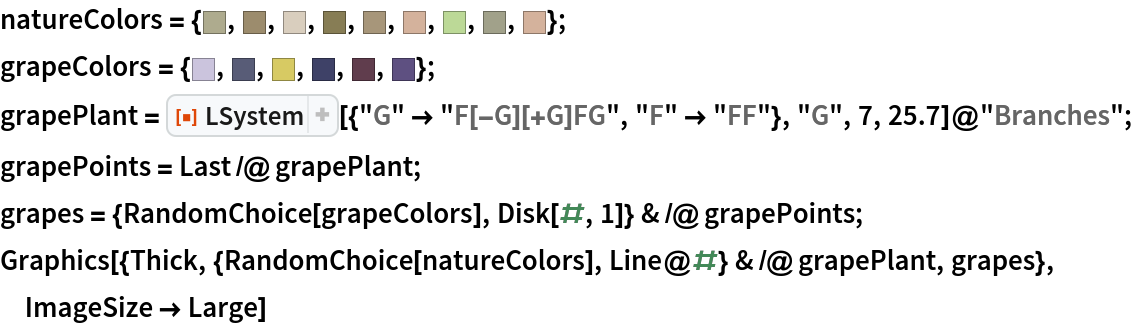Out=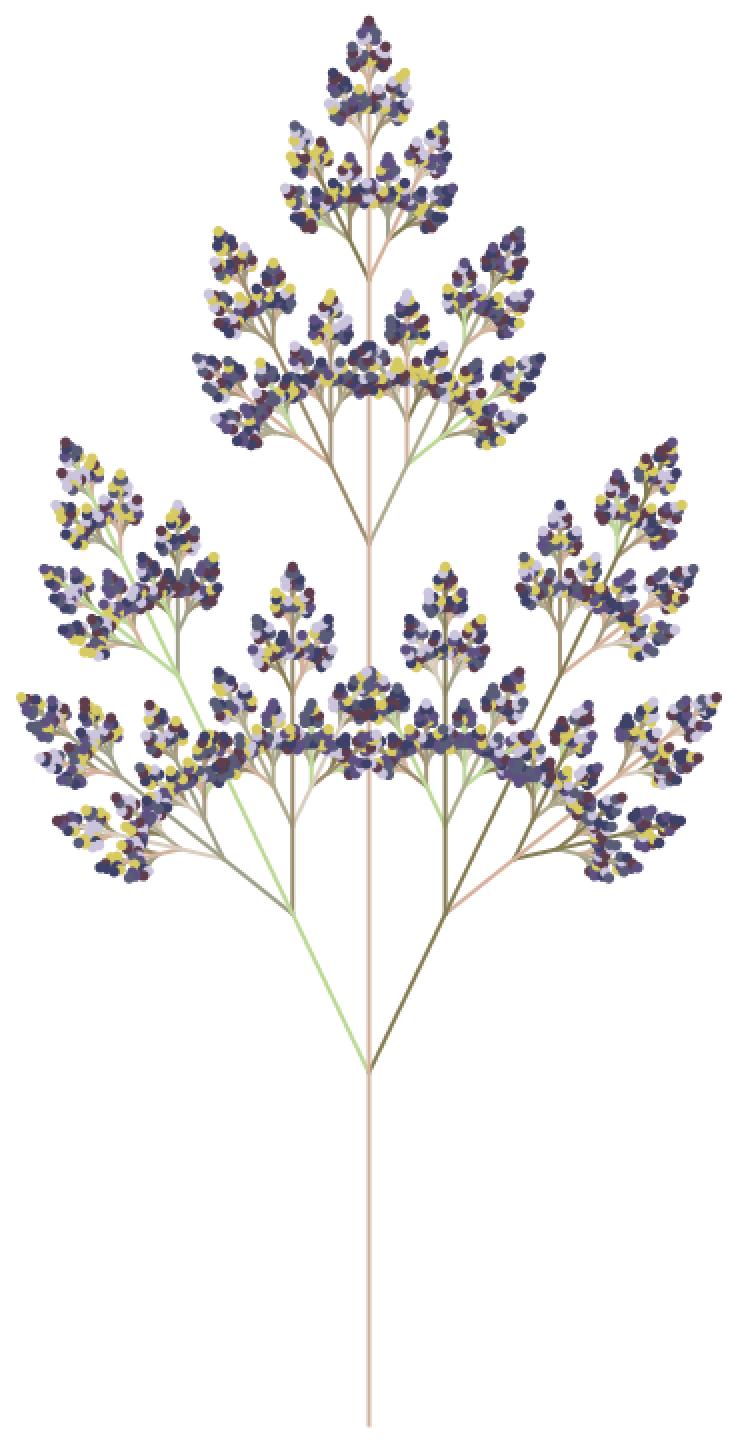### Possible Issues (1)

The execution time tends to grow very fast according to the number of iterations:

 In:=Out=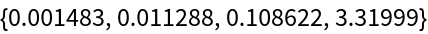In:=Out=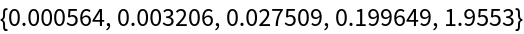### Neat Examples (2)

An animated orange tree:

 In:=In:=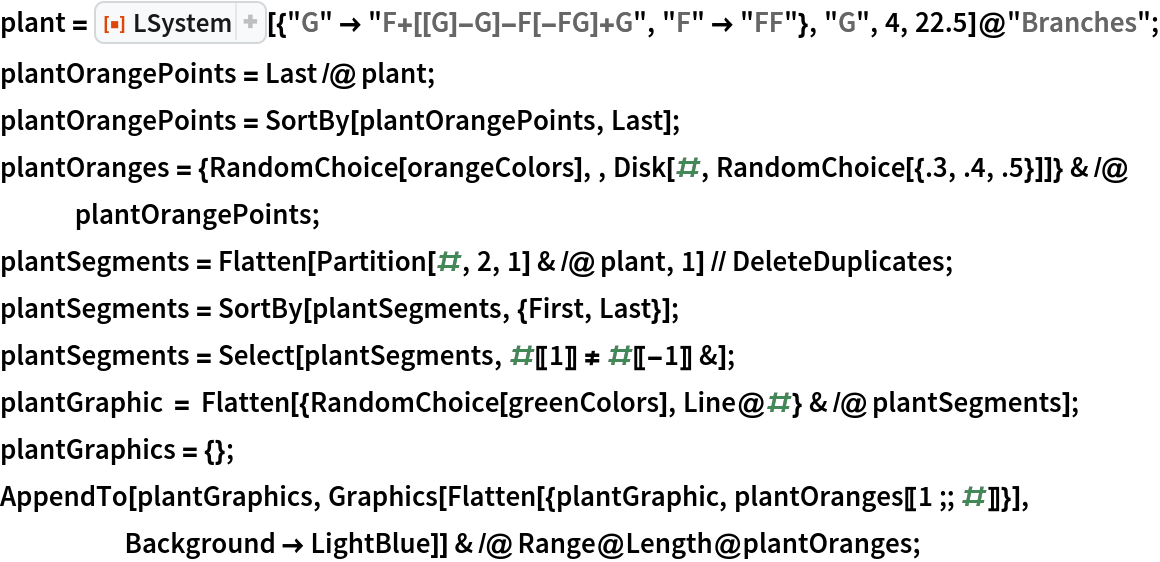In:=Out=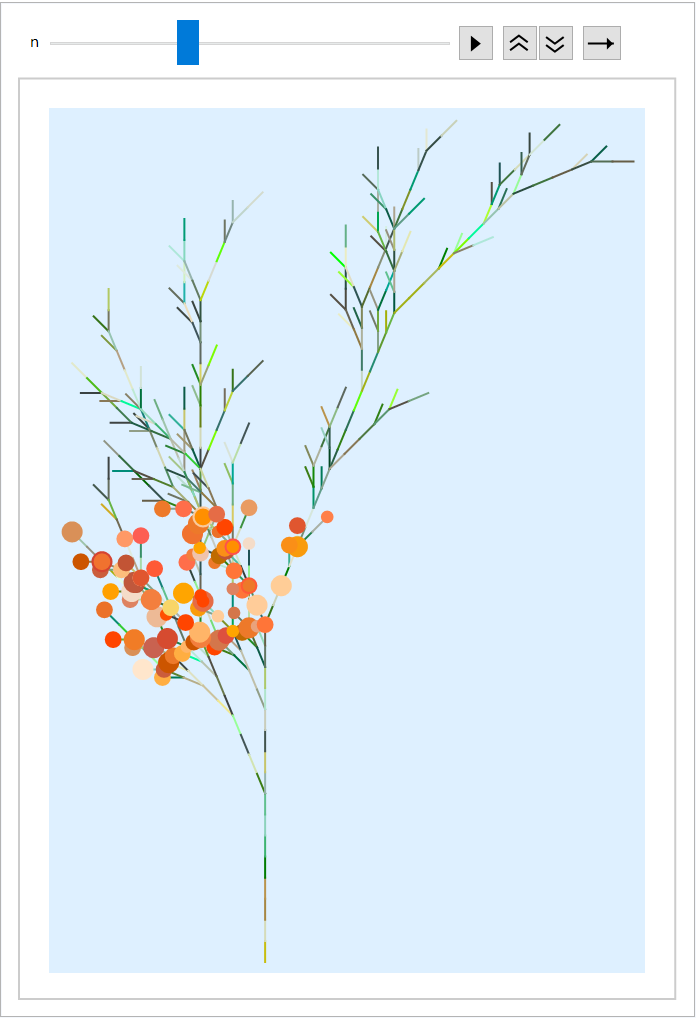Generate a snowflake:

 In:=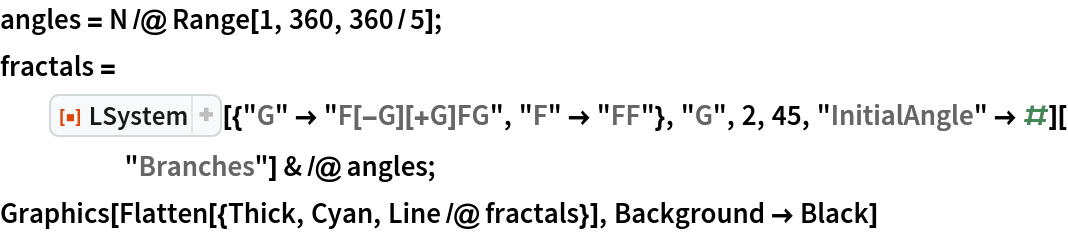Out=